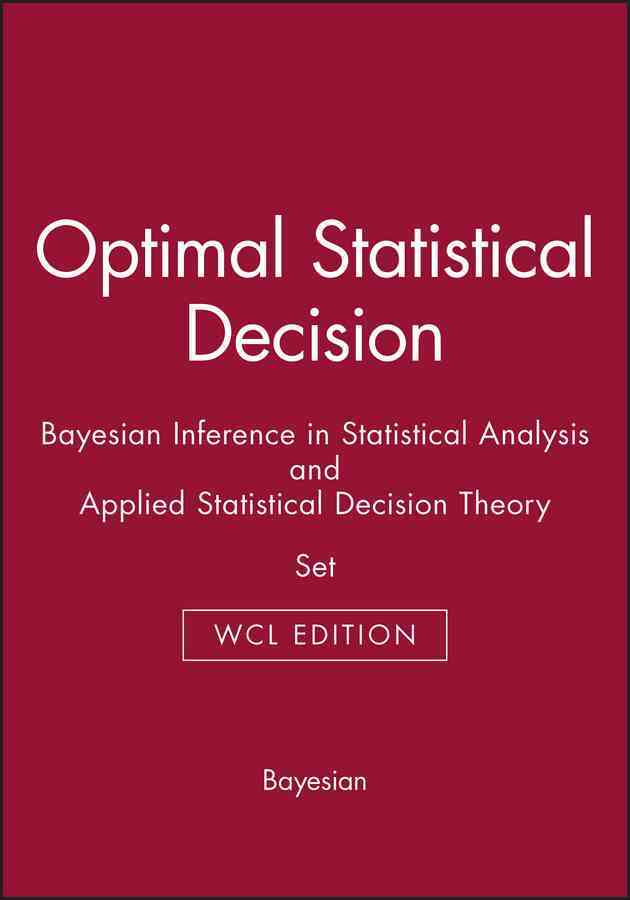# Optimal Statistical Decision & Bayesian Inference in Statistical Analysis & Applied Statistical Decision Theory Morris H. DeGroot ; George E. P. Box ; George C. Tiao ; Howard Raiffa

Set that includes three works covering statistical decision theory and analysis The three books within this set are Optimal Statistical Decisions, Bayesian Inference in Statistical Analysis, and Applied Statistical Decision Theory. Optimal Statistical Decisions discusses the theory and methodology of decision-making in the field. The volume stands as a clear introduction to Bayesian statistical decision theory. A second book, Bayesian Inference in Statistical Analysis, examines the application and relevance of Bayes' theorem to problems that occur during scientific investigations, where inferences must be made regarding parameter values about which little is known. Key aspects of the Bayesian approach are discussed, including the choice of prior distribution, the problem of nuisance parameters, and the role of sufficient statistics. Applied Statistical Decision Theory covers the development of analytic techniques in the field of statistical decision theory. This classic book was first published in the 1960s.

#### Niet leverbaar

Deze titel is (nog) niet op voorraad.

### Specificaties

ISBN
9780471687887
Uitgever
John Wiley & Sons Inc
Druk
1e
Datum
15-08-2006
Taal
Engels
1476 pp.
Bindwijze
Paperback
Genre
Wetenschap

### Koop dit boek bij Athenaeum

• Gratis verzending vanaf € 20,-.
• Bestellen zonder registratie of login.
• Vertrouwde service, veilige afhandeling.